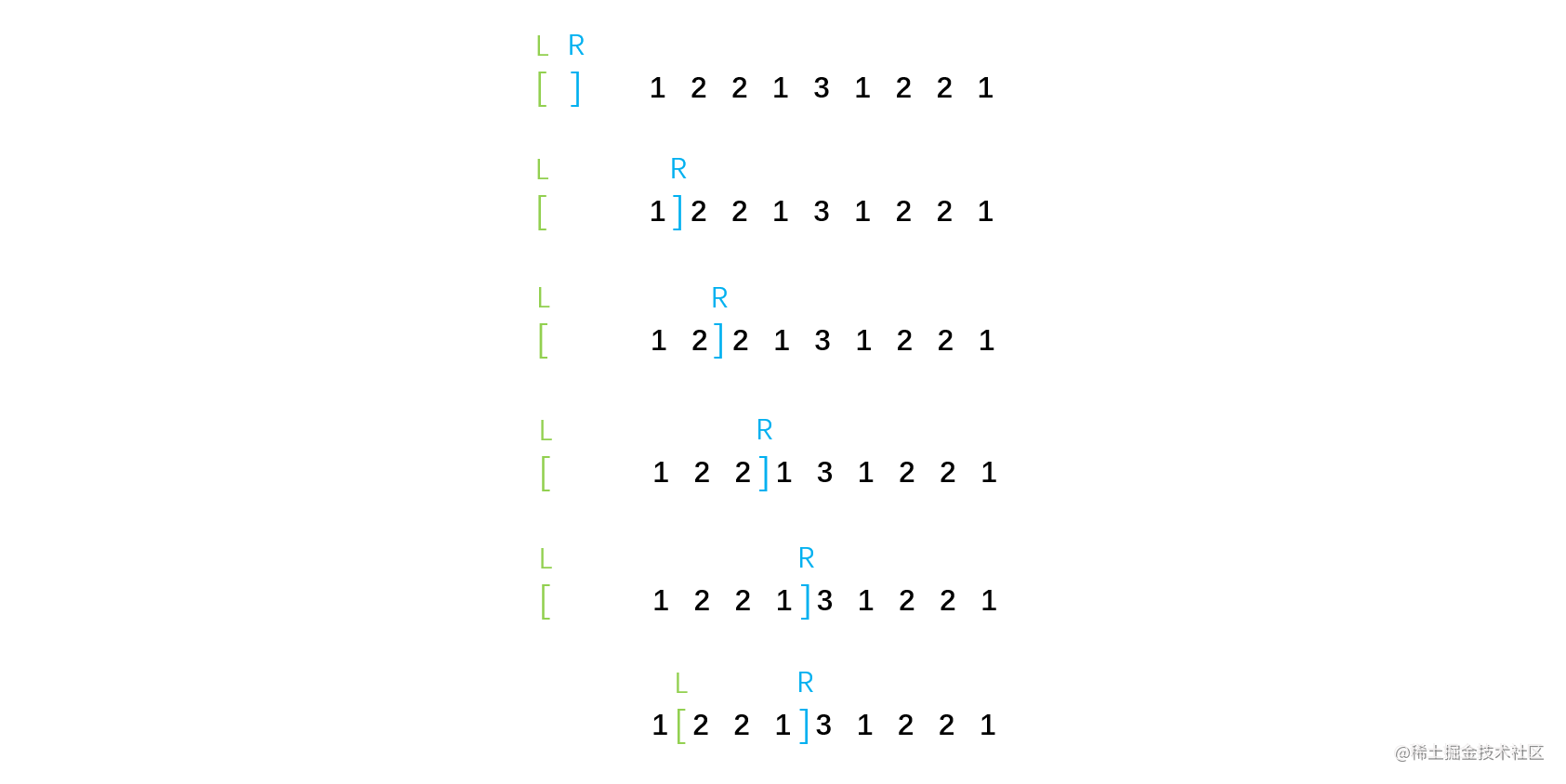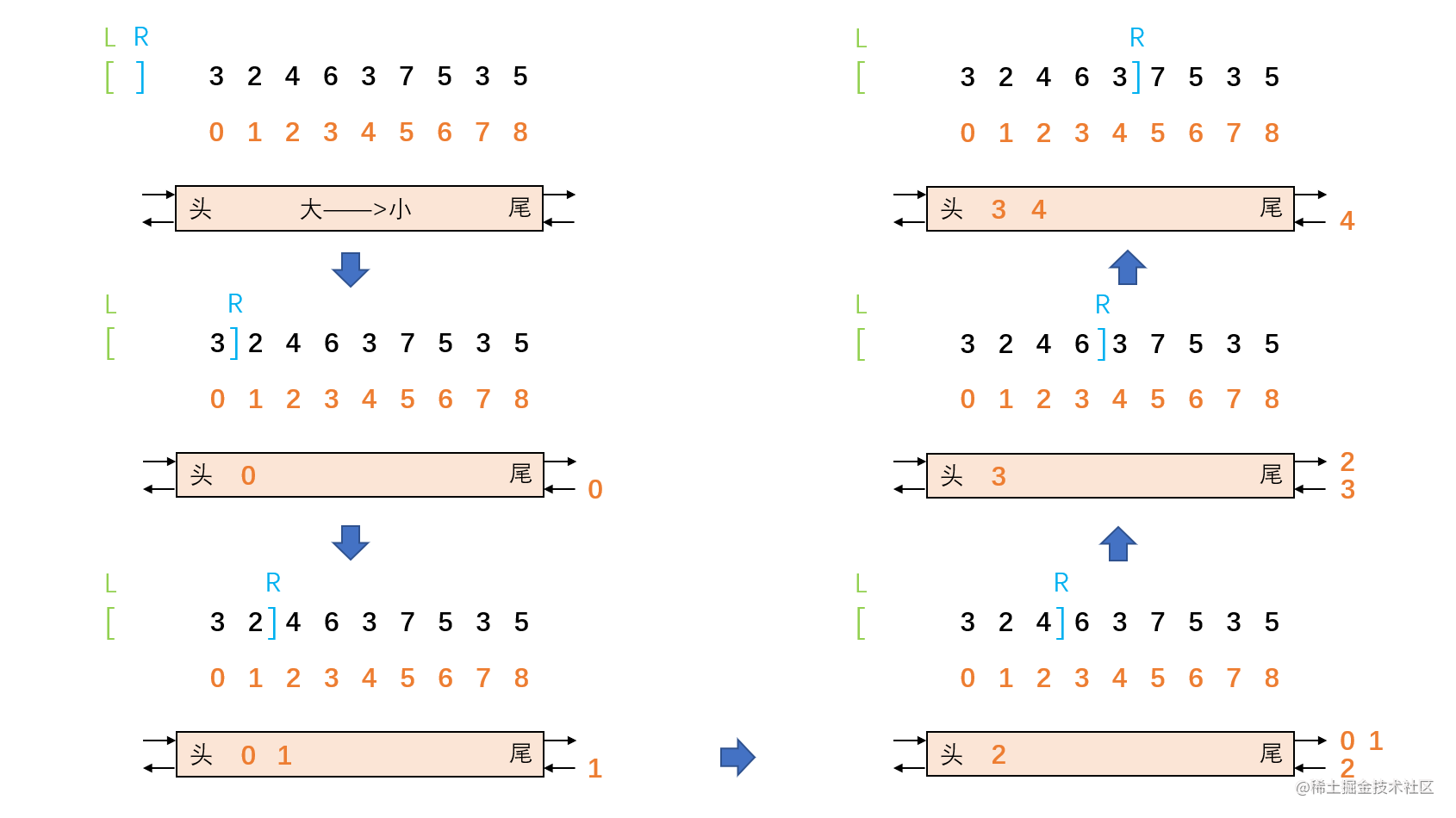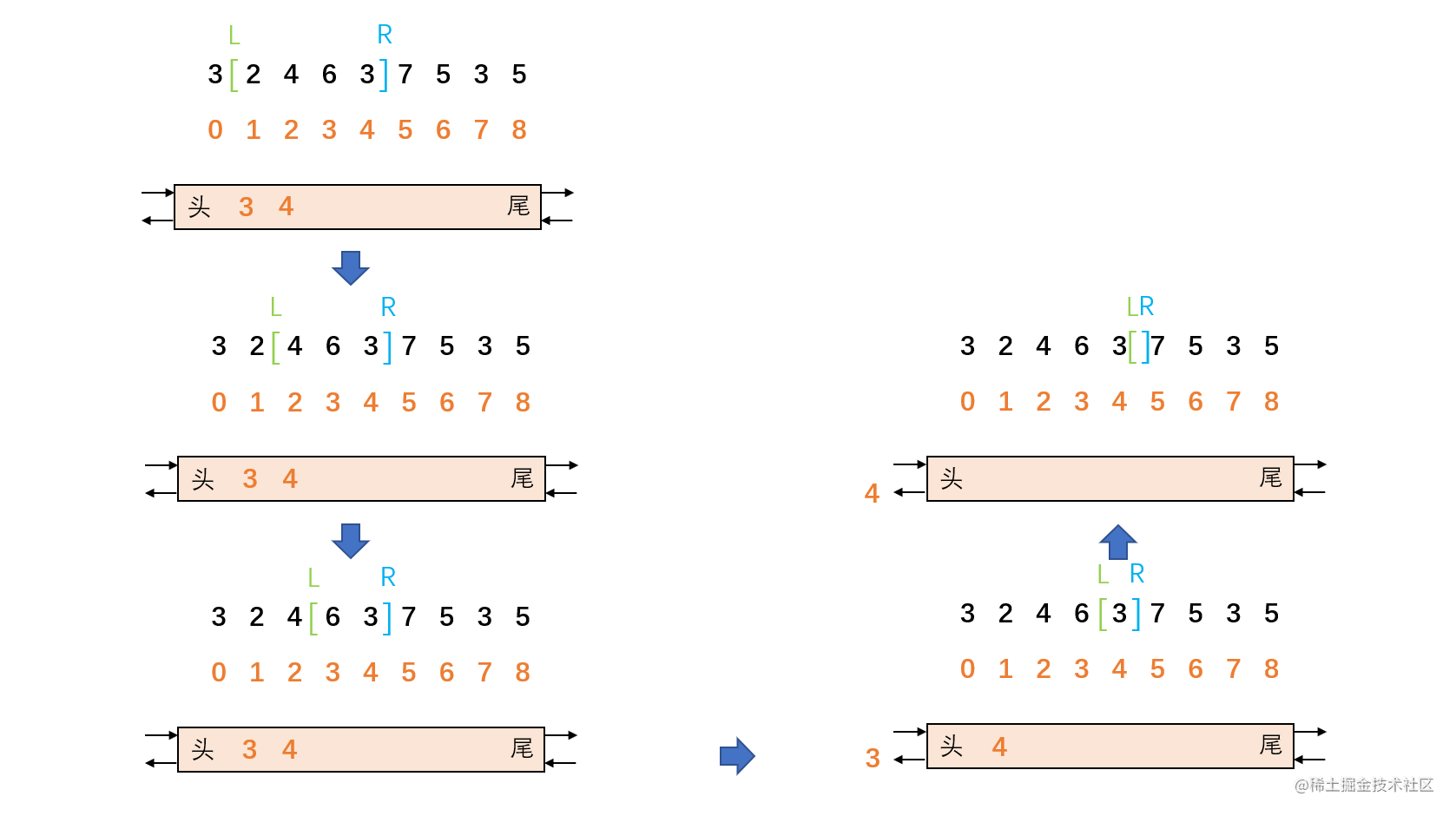# 【数据结构与算法】滑动窗口详解

## 滑动窗口

### 1. 窗口### 2. 引入

[ 4 3 5 ] 4 3 3 6 7 窗口最大值 = 5

4 [ 3 5 4 ] 3 3 6 7 窗口最大值 = 5

4 3 [ 5 4 3 ] 3 6 7 窗口最大值 = 5

4 3 5 [ 4 3 3 ] 6 7 窗口最大值 = 4

4 3 5 4 [ 3 3 6 ] 7 窗口最大值 = 6

4 3 5 4 3 [ 3 6 7 ] 窗口最大值 = 7

### 3. 最大值更新结构

R 向右移动一位：• 如果双端队列为空，那么 R 新囊括的元素的下标从尾部直接进入双端队列。
• 如果双端队列不为空，则 R 新囊括的元素需要与双端对列尾部的下标所指向的元素进行比较：
• 如果新囊括的元素比双端对列尾部下标所指向的元素小，则直接从尾部进入双端对列。
• 如果新囊括的元素比双端对列尾部下标所指向的元素大或者相等，则将尾部的下标从尾部弹出双端队列，让新囊括的元素与当前尾部下标所指向的元素继续比较。

L 向右移动一位：• 只需要将 L 新排出的元素对应的下标和双端队列头部的下标进行比较：
• 如果新排出的元素对应的下标和双端队列头部下标一致，则将头部的下标从头部弹出双端队列。
• 如果新排出的元素对应的下标和双端队列头部下标不一致，无需任何操作。

### 5. 原理3、2 和 1 弹出的原因是 arr = arr > arr > arr，且 arr、arr 和 arr 又一定比 arr 更早过期，所以 arr 、arr 和 arr 再也没有机会成为最大值了，因此可以直接从双端队列中弹出，让 arr 压入双端队列就足够了。

### 7. 实现``````public class SlidingWindow {

// 窗口左边界
private int left;

// 窗口右边界
private int right;

// 窗口滑动的数组
private int[] arr;

public SlidingWindow(int[] arr) {
this.left = -1;
this.right = -1;
this.arr = arr;
}

// 窗口左边界向右滑动n个单位
public void leftSlide(int n) {
// 如果left和right交相交或者left越界，则滑动失败
if (this.left + n > this.right) {
return ;
}

// left向右滑动n个单位
for (int i = 0; i < n; i ++) {
this.left ++;
// 如果left新排出的下标和队首下标相同，则队首下标出队列
if (left - 1 == queue.getFirst()) {
queue.pollFirst();
}
}
}

// 窗口右边界向右滑动n个单位
public void rightSlide(int n) {
// 如果right越界，则滑动失败
if (this.right + n >= arr.length) {
return ;
}

// right向右滑动n个单位
for (int i = 0; i < n; i ++) {
this.right ++;
// 如果right新囊括的下标对应的元素大于等于队尾下标对应的元素，队尾下标出队列
while (!queue.isEmpty() && arr[right] >= arr[queue.getLast()]) {
queue.pollLast();
}
// right进队列
}
}

// 获取当前窗口内的最大值
public int getMax() {
// 如果当前双向队列为空
if (this.queue.isEmpty()) {
return -1;
}

// 获取对头下标对应的元素
return arr[this.queue.peek()];
}

}

### 8. 解题

[ 4 3 5 ] 4 3 3 6 7 窗口最大值 = 5

4 [ 3 5 4 ] 3 3 6 7 窗口最大值 = 5

4 3 [ 5 4 3 ] 3 6 7 窗口最大值 = 5

4 3 5 [ 4 3 3 ] 6 7 窗口最大值 = 4

4 3 5 4 [ 3 3 6 ] 7 窗口最大值 = 6

4 3 5 4 3 [ 3 6 7 ] 窗口最大值 = 7

``````public static int[] getSlidingWindowMax(int[] arr, int w) {
int[] res = new int[arr.length - w + 1];

SlidingWindow slidingWindow = new SlidingWindow(arr);

// right先向右走w步
slidingWindow.rightSlide(w);
// left先向右走1步，此时窗口范围是 0 ~ (w-1)，窗口大小就是w
slidingWindow.leftSlide(1);

// 获取每一个窗口状态的最大值
for (int i = 0; i < res.length; i ++) {
res[i] = slidingWindow.getMax();

// 窗口整体向右移动1位
slidingWindow.rightSlide(1);
slidingWindow.leftSlide(1);
}

return res;
}